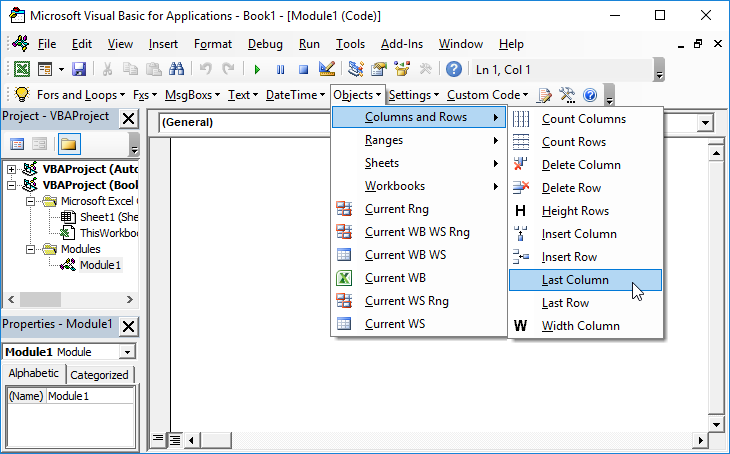# VBA – Determine a Variable’s Underlying Type

## Determine VBA Variable Type

Use TypeName(variable name) after setting the variable equal to something” Many thanks to Mark Bigelow.

This could come in very handy if you’re ever sitting and scratching your head over a “type mismatch” error.

The following example demonstrates the use of TypeName to show the underlying type changes of a Variant.

``````

Sub WhatIsTheType()

Dim Mark As Variant

Mark = 1

MsgBox Mark & " makes the variable " & TypeName(Mark)

Mark = 111111

MsgBox Mark & " makes the variable " & TypeName(Mark)

Mark = 111111.11

MsgBox Mark & " makes the variable " & TypeName(Mark)

End Sub

``````